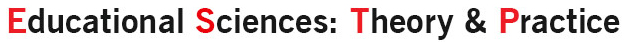Educational Sciences: Theory & Practice

## ISSN: 2630-5984## Investigating Test Equating Methods in Small Samples Through Various Factors

##### Semih Aşiret
Department of Measurement and Evaluation in Education, Faculty of Education, Mersin University, Yenişehir Campus, Mersin 33169 Turkey
##### Seçil Ömür Sünbül
Department of Measurement and Evaluation in Education, Mersin University, Mersin 33169 Turkey

## Abstract

In this study, equating methods for random group design using small samples through factors such as sample size, difference in difficulty between forms, and guessing parameter was aimed for comparison. Moreover, which method gives better results under which conditions was also investigated. In this study, 5,000 dichotomous simulated data consistent with the three-parameter logistic model were produced for each form (X and Y) by manipulating the factors’ levels for random group design. In order to equate two test forms, the random groups design was used in this study. Simulated test forms were equated using the equating methods of identity, mean, linear, circle-arc, and 2- and 3-moments pre-smoothed equipercentile for different sample sizes (10, 25, 50, 75, 100, 150, 200) through 100 replications. The results obtained from this simulation study were evaluated based on the criterion of equating error (RMSE). The findings indicated that in the case where the sample size was 50 or more and the difficulty difference between forms was 0.4, equating the forms was concluded to give better results than not equating. Moreover, the circle-arc and mean-equating methods produced lower equating errors than other equating methods for small-sample equating under most of the conditions studied.

##### Keywords
Test equating, Equating error, Small samples.